# Startup Jungle Cambodia | How to Build your First Machine Learning Application

Dec. 12, 2017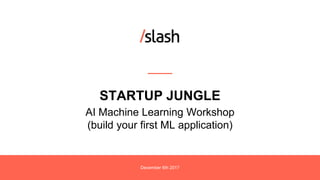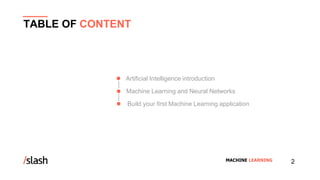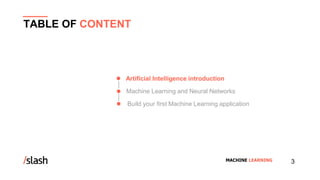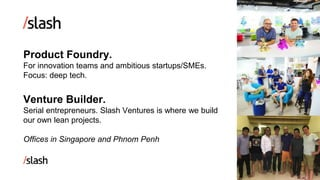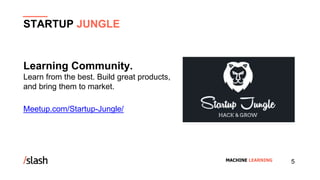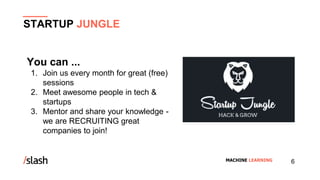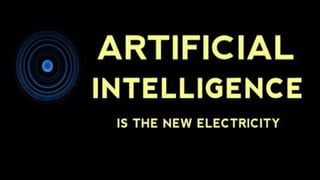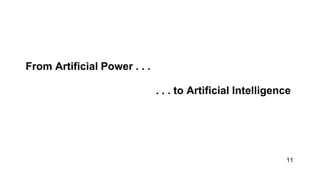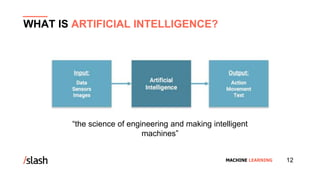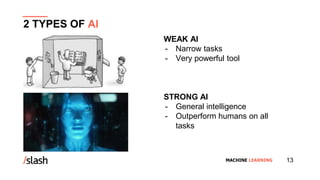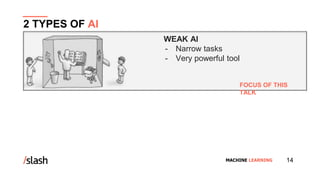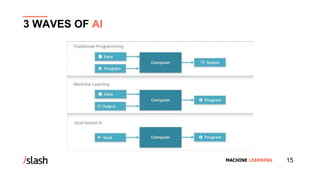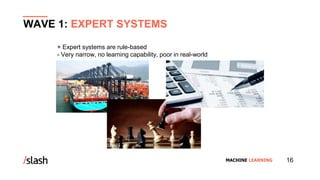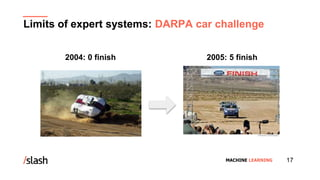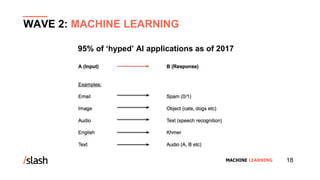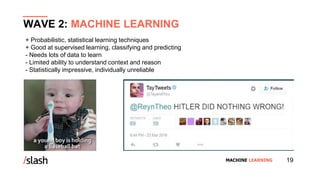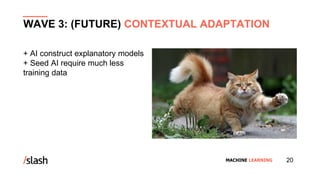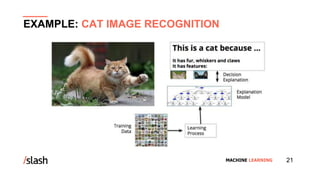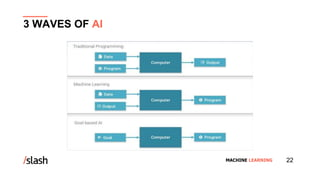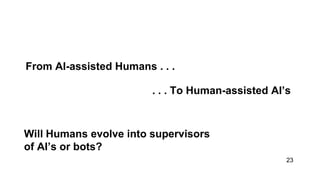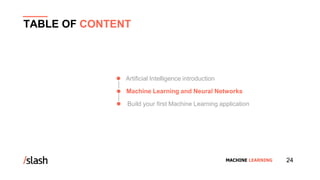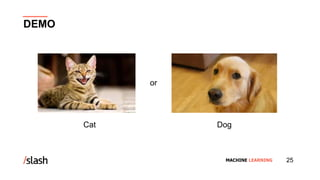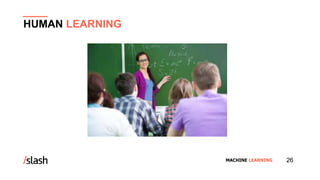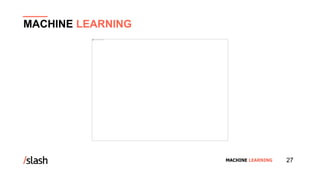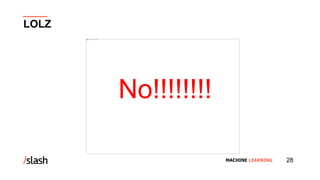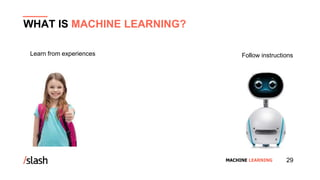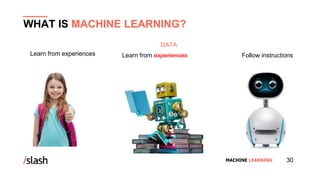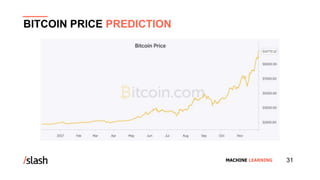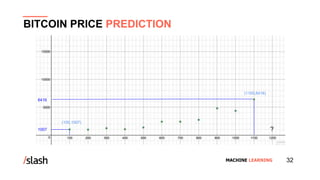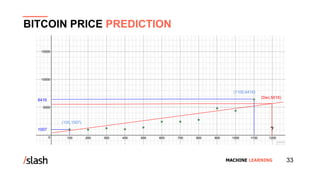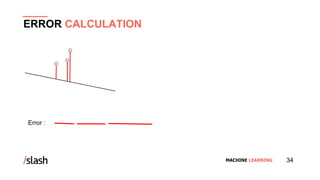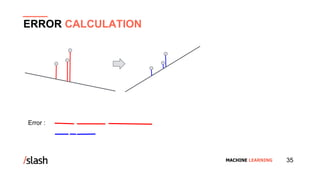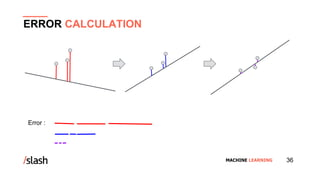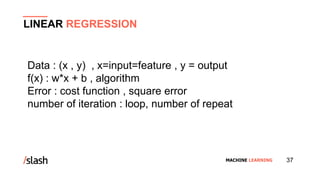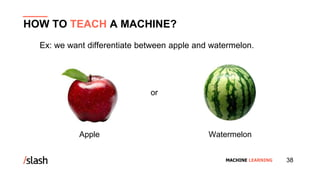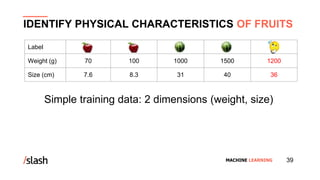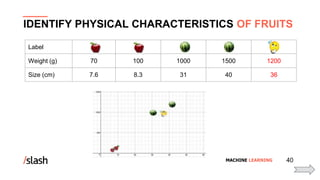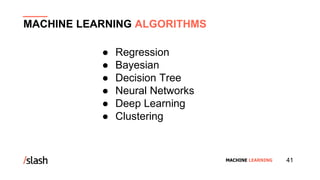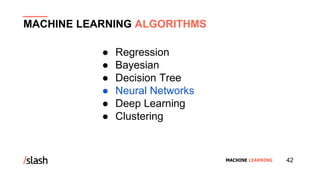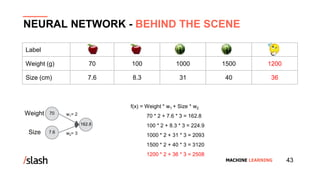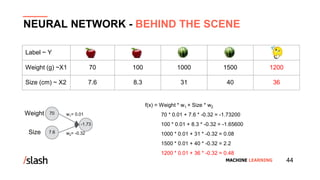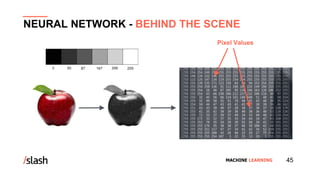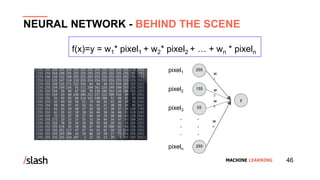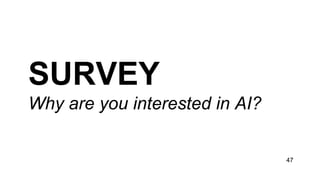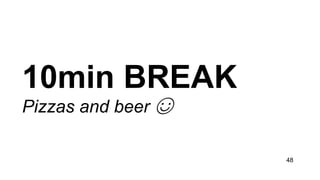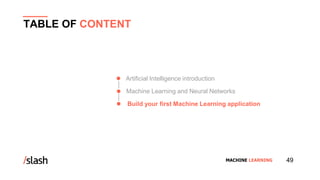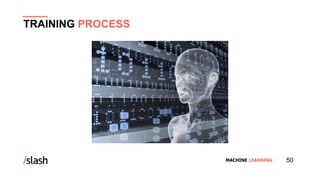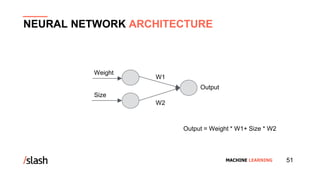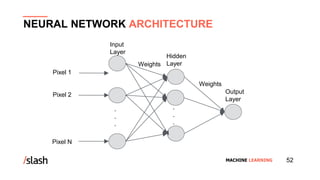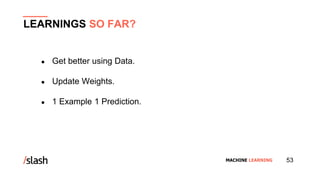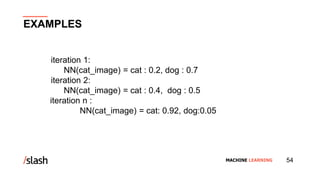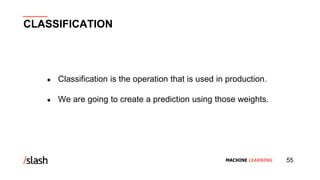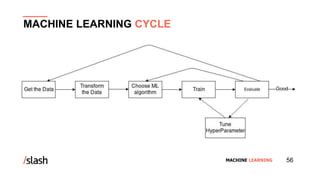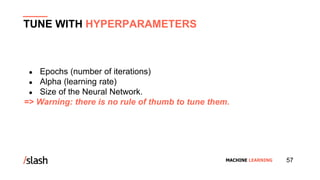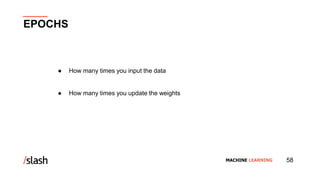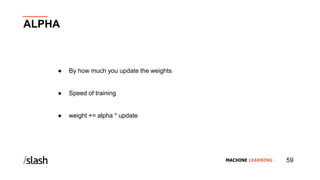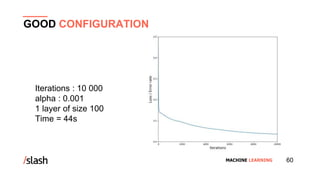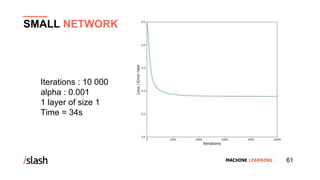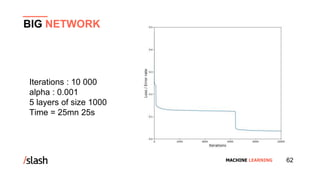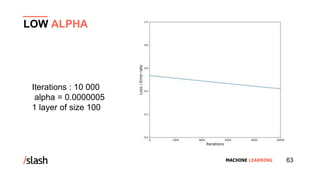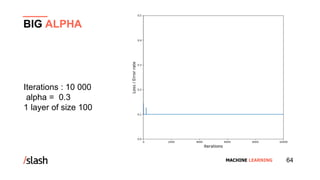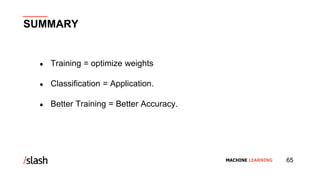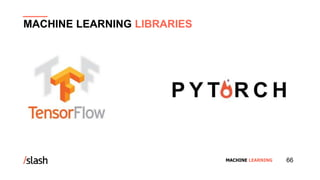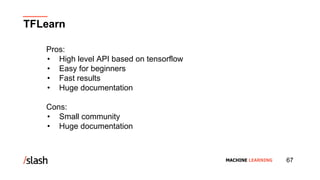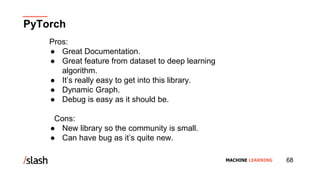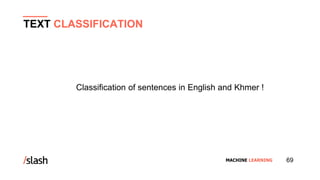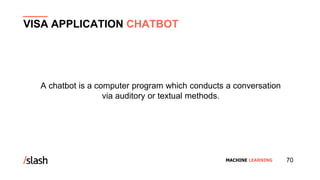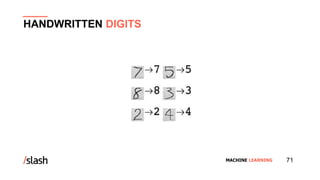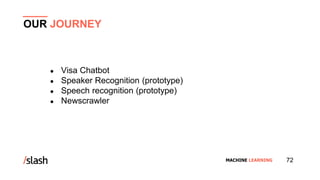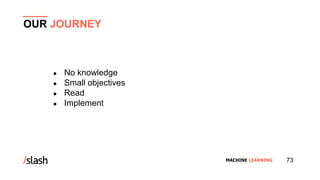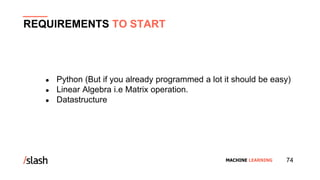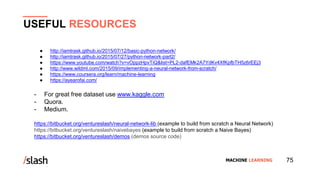1 of 75

### Editor's Notes

1. What is Machine Learning & its process. Team members introduction
2. Simple Gathering data Preparing data Choosing models Train Evaluation Hyperparameter Tuning Prediction
3. What the main different between human and machine learning? Here is the human learning, we have a beautiful lady is teaching her students.
4. In this image you will see a very cute machine teacher who is teaching his machine students. Is that call machine learning ?
6. So what is the machine learning. This is the real world human and machine, what is the main differences between human and machine is , human learn from past experiences while machine need to tell what to do, they need to be programed, they follow the instructions. Another question is, can we can get machine to learn from past experience too? The answer yes we can. That’s what machine learning is .
7. for machines, the past experiences have a name , we called data. So in the next few minutes, i’m going to show you few examples in which we can teach machine to learn from previous data, more importantly i’m going to show you that, these algorithms are actually pretty easy and the machine learning is nothing to fear.
8. https://www.desmos.com/calculator/crzk7slwsb This example you cannot apply for investing bitcoin, unless you need to research more about it. You can improve it later Let’s have a look the first example, let’s say we studying the bitcoin price market, our task is to predict a price of the bitcoin by given the date. The graph is the price of the bitcoin in 2017 from January to November. Now we want to predict a price in December. How can we do?
9. To help us out we collect some previous data of the bitcoin price,Let put data in the grid, the x-axis represents the date of the bitcoin’s price and the y-axis represents the price of the bitcoin in dollars.. To make it easy and calculable I transform the date to numbers. So 100 represent as the month of January and 200 represent as February and so on. Can you guess the bitcoin price in december or (equal to 1200) by given these data? What is the best estimate for the price of the bitcoin? 5000, 6000 or 7000 ?
10. to help us out we can see the green points kind of form a line so we can draw a line that best fit data. So we can draw a line that best fit the data. Now in this line we can say our best guess for the price of bitcoin in December is 5616\$. You may ask how can i find this line.
11. Let look at simple example these three points, we are going to try to find the best fit line that fit with those three points. Since we are teaching computer how to do it , computer cannot eyeball the line so you have to get it to draw a random line and see how bad of this line is. In order to see how bad of this line is. We calculate the error. So we gonna calculate the error look at the lengths of the distances from the line to these three points. We are going just to simply say that error is the sum of those red lines.
12. What we gonna to do is next, move line around and see if we can reduce the error. Assuming we move the line to this direction and we calculate the error. And we see the error become smaller and smaller.
13. Let say we take that step to closer our solution. If we continue doing this procedure several times, we always decreasing the error and we will finally arrive to a good solution in the form of this line. https://www.desmos.com/calculator/2zunvwck21 https://www.desmos.com/calculator/0dej2z5luc
14. In data , we have x and y , x is the date of the bitcoin price we called input or feature , and y is bitcoin price we called an output. every machine learning algorithm , it has its own formula or we can called function. In the formula, it consist of weight and bias, To make our formula or algorithm more accurate, we need to have a good weight value and bias value. To judge it is a good weight and bias or not , we need to calculate the error or we called cost function or square error. To try several time to find a good weight we need to set number of iteration or a loop, how many time we need to repeat and find a good weight.
15. Assuming i collect 4 fruits from the market, 2 are apple and 2 are watermelon, i weight and size all the fruits and i note it in the table. And my mom buy me another fruit, but i don’t know what the fruit is, she just gave me the weight and size of the fruit, and i try to guess what is that fruit. To guess it , i try to observe the number that i’ve already weight and size it. I notice that , watermelon seems to be bigger and heavier. So now i am able to guess now, it should be a watermelon. This technique is not working for the big dataset. We cannot observe hundred of data points.
16. Let try another technique , you take all of those data points that i was collected. And plot it in the graph. With this graph you will able to guess or predict very quickly , that is a watermelon. Because the new data is close to watermelon. So now how to find an algorithm to solve with this problem?
17. Let talk a little bit with some machine learning algorithm, We have a lot of algorithms out there. Here are some of those.
18. Now we choose Neural Networks to present today. You may ask, why we choose Neural Networks to solve the problem? Because it works like a human brain. Neural Networks are the biologically inspired simulations performed on the computer to perform certain specific tasks like clustering, classification, pattern recognition etc. Some examples of the pattern are — fingerprint image, a handwritten word, human face or speech signal.
19. https://www.desmos.com/calculator/xezilnxrbz https://www.desmos.com/calculator/coknirwubg
20. Back to the slide 38 and 39, If you go further for the machine learning, all input data is digital, we cannot weigh and size by image with those digital data, so we need to extract the feature from the image that is called pixel value.
21. Each pixel belong to one node
22. Reminder of what kevin’s said And explain that this kind of network is not sufficient
23. Explain that the hidden layer is used to have more learnable parameter More than one hidden layer is possible We will explain how to configure that
24. https://towardsdatascience.com/the-7-steps-of-machine-learning-2877d7e5548e Explain about evaluate and training set
25. How long will be your training
26. Visa chatbot using neural networks from scratch. Better to support Khmer language. Mor dataset for accuracy Speaker from scratch and with pytorch same result ~70% accuracy. Speech Recognition from scratch. NewsCrawler using semi-supervised learning and naive bayes(not neural network but use probability laws). It’s good to propose the interesting articles to the users.
27. From no knowledge began with some really really easy tutorial and tried to code a neural network from scratch. Read many articles and implement them at the same time. One thing we did not do but can be great use kaggle dataset and try to “solve it”
28. Python is easy to learn. It has many libraries for machine learning. Linear Algebra Matrix Operation or transformation Vectors and spaces Alternate Coordinate Systems